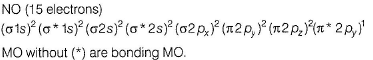Courses

# Test: Molecular Orbital Theory

## 23 Questions MCQ Test Chemistry Class 11 | Test: Molecular Orbital Theory

Description
This mock test of Test: Molecular Orbital Theory for JEE helps you for every JEE entrance exam. This contains 23 Multiple Choice Questions for JEE Test: Molecular Orbital Theory (mcq) to study with solutions a complete question bank. The solved questions answers in this Test: Molecular Orbital Theory quiz give you a good mix of easy questions and tough questions. JEE students definitely take this Test: Molecular Orbital Theory exercise for a better result in the exam. You can find other Test: Molecular Orbital Theory extra questions, long questions & short questions for JEE on EduRev as well by searching above.
QUESTION: 1

### Direction (Q. Nos. 1-14) This section contains 14 multiple choice questions. Each question has four choices (a), (b), (c) and (d), out of which ONLY ONE option is correct. Q. Assuming that Hund’s rule is violated, the bond order and magnetic nature of the diatomic molecule B2 is [IIT JEE 2010]

Solution:

B2 (10 electrons)
MO electronic configuration is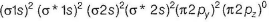Bonding electrons = 6
Anti-bonding electrons = 4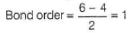No unpaired electron-diamagnetic

QUESTION: 2

### In which of the following pairs of molecules/ions both the species are not likely to exist?

Solution:

Species with (zero) bond order will not exist.
Electrons in orbital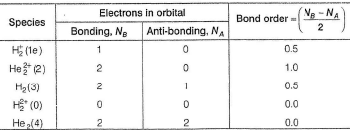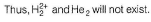QUESTION: 3

### Among the following the maximum covalent character is shown by the compound

Solution:
QUESTION: 4

Assuming (2s-2p) mixing is not operative, the paramagnetic species among the following is

Solution:

If (2s-2p) mixing is not operative, then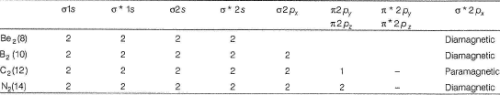QUESTION: 5

Select the correct statem ent about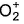and and O2.

Solution: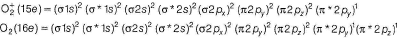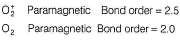QUESTION: 6

The common features among the species CN-, CO, NO+ and N2 are

Solution: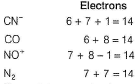Thus, all these are isoelectronic. MO electronic configuration is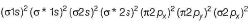No electron unpaired - diamagnetic
Bonding electrons = 10
Anti-bonding electrons = 4
Thus, bond- order =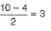QUESTION: 7

According to MO theory which of the following lists ranks the nitrogen species in terms of increasing bond order?

Solution: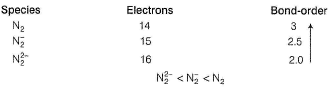QUESTION: 8

O — F and F2 can be compared in terms of

Solution: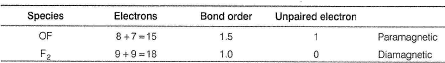Bond energy of O — F (1.5) > F — F (1)
Thus, OF is more stable than F2 thus, (c).

QUESTION: 9

Which of the following diatomic molecules would be stabilised by the removed of an electron?

Solution:

A molecule is stabilised if bond-order increases, an anti-bonding electron is lost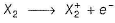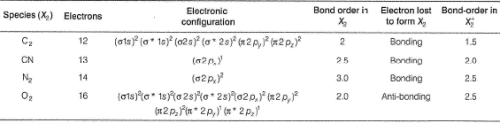QUESTION: 10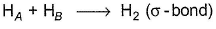Probability (electron charge density) of bonding and anti-bonding molecular orbitals are given.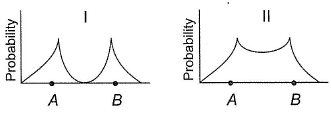Select the correct probability,

Solution:

Electron-charge density in a bonding molecular orbital is high in the internuclear region as shown in II.
In an anti-bonding molecular orbital, it is high in parts of the molecule away from the internuclear region.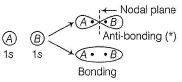QUESTION: 11

If one of the electrons in the He2 molecule is taken to the next excited state, then bond order in He2

Solution: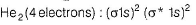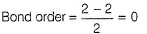Electron is taken to next excited state that is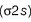hen electronic configuration is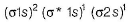Number of electrons in bonding molecular orbital = 3
and in anti-bonding molecular orbital = 1
Thus, bond order = (3 - 1) /2 =1
Thus, bond order increases by 1 unit.

QUESTION: 12

If one of the electrons (1s2) of helium is taken in excited state then bond order of He2 is

Solution: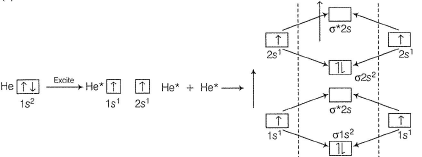Number of electrons in bonding orbital = 4 and in anti-bonding orbitai = 0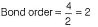QUESTION: 13

The bond energy of H2 is 436 kJ mol -1. Thus, bond energy of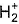is

Solution: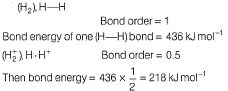QUESTION: 14

Consider the following oxidation/reduction process,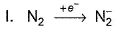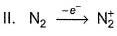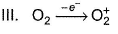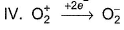Q. Magnetic moment does not change in

Solution: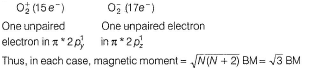QUESTION: 15

Direction (Q. Nos. 15) This sectionis based on statement I and Statement II. Select the correct answer from the code given below.

Q.

Statement I : N2 has a greater dissociation energy than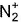, where as O2 has lower dissociation energy than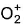.

Statement II : N2 has 14 electrons while O2 has 16 electrons .

Solution: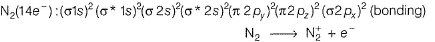Electron from bonding molecular orbital of higher stability is lost (requires higher energy).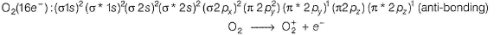Electron from antibonding molecular orbital of lower stability is lost (requires lower energy).
N2 has 14 electrons and O2 has 16 electrons.
Thus, both Statements I and II are correct but Statement II is not the correct explanation of Statement I

*Multiple options can be correct
QUESTION: 16

Direction (Q. Nos. 16-18) This section contains 4 multiple choice questions. Each question has four choices (a), (b), (c) and (d), out of which ONE or  MORE THANT ONE  is correct.

Q. Set of species with identical bond order is/are

Solution:

isoelectronic species will generally have identical bond order: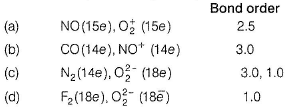*Multiple options can be correct
QUESTION: 17

One of the electrons of the highest energy level is taken to next excited state in the following diatomic species. Select the species which undergoes change in bond order?

Solution: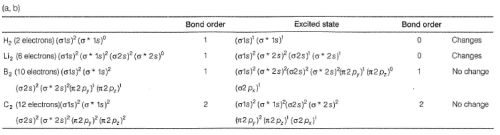*Multiple options can be correct
QUESTION: 18

In which of the following processes, does the value of magnetic moment change ?

Solution:

(a) CO (14e), no unpaired electron, magnetic moment = 0
CO+ (13e), One electron is lost from bonding melecular orbital unpaired electron = 1, magnetic moment =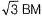(b)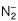(15e) Unpaired electron = 1, magnetic moment =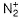(13e) Unpaired electron = 1, magnetic moment =(c) Zn, Zn2+, unpaired electron = 0
(d) O2 (16e), unpaired electron = 2, magnetic moment =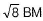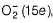, unpaired electron = 1, magnetic moment =QUESTION: 19

Direction (Q. Nos. 19-20) This section contains  a paragraph, each describing theory, experiments, data etc. three Questions related to paragraph have been given.Each question have only one correct answer among the four given options (a),(b),(c),(d)

Valence shell MO electronic configuration of a diatomic species is shown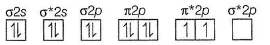* is for anti-bonding molecular orbital (MO).

Q. Bond order of this species is

Solution:

Total number of electrons = 16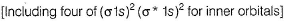Number of bonding electrons = 10,
Number of anti-bonding electrons = 6,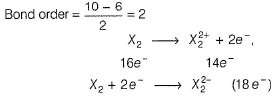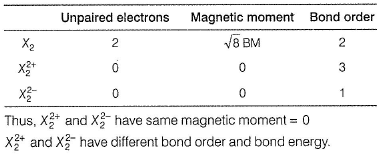QUESTION: 20

Valence shell MO electronic configuration of a diatomic species is shown* is for anti-bonding molecular orbital (MO).

Q. Divalent cation of this species

Solution:

Total number of electrons = 16Number of bonding electrons = 10, Number of anti-bonding electrons = 6,QUESTION: 21

Direction (Q. Nos. 21) Choice the correct combination of elements and column I and coloumn II  are given as option (a), (b), (c) and (d), out of which ONE option is correct.

Q. Match the conversion in Column l with the type of effect given in Column II.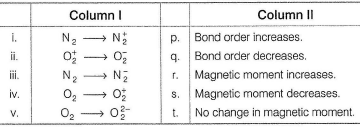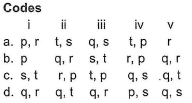Solution: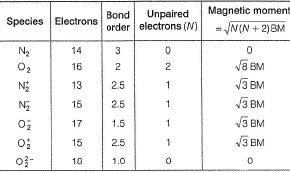Thus, (i) - (q , r)    (ii) - (q.t),
(iii) - (q,r),    (iv)- (P.s).
(v) - (q,s).

*Answer can only contain numeric values
QUESTION: 22

Direction (Q. Nos. 22 and 23) This section contains 3 questions. when worked out will result in an integer from 0 to 9 (both inclusive).

Q. Total number of electrons in anti-bonding MO in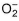(superoxide ion) is .......

Solution:(17 electrons) has molecular orbital electronic configuration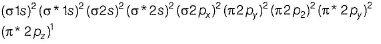Underlined are anti-bonding molecular orbital Thus, seven electrons are in anti-bonding molecular orbitals.

*Answer can only contain numeric values
QUESTION: 23

How many bonding MO are used in the formation of NO?

Solution: